# Round flowerbed

Around a round flowerbed with a diameter of 6 meters and I will make a sidewalk up to 0.5 meters wide. How much gravel is needed if the layer is to be 5 cm high?

V =  0.5105 m3

### Step-by-step explanation: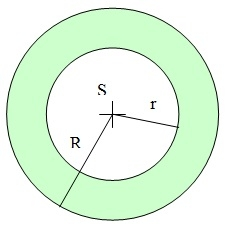Did you find an error or inaccuracy? Feel free to write us. Thank you!Tips to related online calculators
Tip: Our volume units converter will help you with the conversion of volume units.

#### You need to know the following knowledge to solve this word math problem:

We encourage you to watch this tutorial video on this math problem:

## Related math problems and questions:

• Around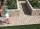Around the circular flowerbed with a radius of 2 m is a sidewalk 80 cm wide. How many square meters does the sidewalk have?
• Sand pathHow many m3 of sand is needed to fill the 1.5m wide path around a rectangular flowerbed of 8m and 14m if the sand layer is 6cm high?
• SidewalkThe city park is a circular bed of flowers with a diameter of 8 meters. Around it, the whole length is 1-meter wide sidewalk. What is the sidewalk area?
• Gravel - cone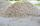The mound of gravel has a regular circular cone shape with a height 3.3 meter and a base circumference of 18.85 meters. How many cubic meters of gravel is in a pile? Calculate the weight of gravel if its density is p = 640 kg / cubic m.
• The hollow cylinderThe hollow cylinder has a height of 70 cm, an outer diameter of 180 cm, and an inner diameter of 120 cm. What is the surface of the body, including the area inside the cavity?
• Circular lawnAround a circular lawn area is 2 m wide sidewalk. The outer edge of the sidewalk is curb whose width is 2 m. Curbstone and the inner side of the sidewalk together form a concentric circles. Calculate the area of the circular lawn and the result round to 1
• Circular flowerbed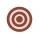Circular flowerbed with diameter 8 m we split by concentric circle to circle and annulus with the same area. Find the radius of the circle.
• LidWhat is the weight of concrete cover (lid) to round shape well with a diameter 1.8 m, if the thickness of the cover is 11 cm? 1 m3 of concrete weighs 2190 kg.
• The pipe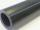The pipe is 1.5 m long. Its outer diameter is 60 cm, inner diameter is 52 cm. Calculate the pipe's weight if the material's density from which it is made is 2 g/cm3. Round the results to whole kilograms.
• Rotary cylinder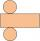The rotating cylinder has a surface area of 69.08 cm2. The area of the shell is 62.8 cm 2. What is the diameter of the cylinder?
• Metal tubeCalculate the metal tube mass 8dm long with the outer radius 5cm and the inner radius 4.5cm and 1cm3 of this metal is 9.5g.
• The collar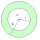The collar on the dress has the shape of an annulus 6 cm wide. The circumference of the inner circle is 31.4 cm. How much cm2 of fabric is needed to make one collar?
• Equilateral cylinderEquilateral cylinder (height = base diameter; h = 2r) has a volume of V = 199 cm3 . Calculate the surface area of the cylinder.
• Steel tubeThe steel tube has an inner diameter of 4 cm and an outer diameter of 4.8 cm. The density of the steel is 7800 kg/m3. Calculate its length if it weighs 15 kg.
• CostumeDenisa is preparing for a goldsmith's costume carnival. During the preparations, she thought she would let her hair wipe instead - she would apply a 5 μm thick layer of gold to each hair. How much gold would Denisa need? Assume that all hundred thousand D
• Sidewalk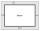In the garden, a 1-meter-wide sidewalk will be paved with tiles around the block-shaped pool. In the picture, the sidewalk is shown in gray. The dimensions of the bottom of the pool are 8.5 meters and 6 meters. The height of the pool walls is 2 meters. Ho
• Garden pondConcrete garden pond has bottom shape of a semicircle with a diameter 1.7 m and is 79 cm deep. Daddy wants make it surface. How many liters of water is in pond if watel level is 28 cm?